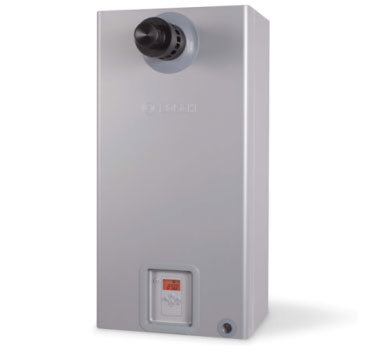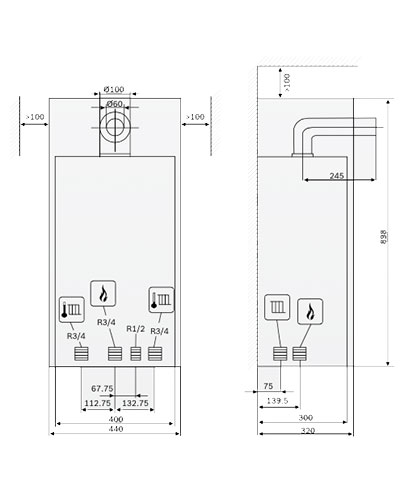< Back# Bosch WBN 6000-30 NG

Maximum
rated heat
output
(Pmax) 80/60ºC
(kW)

Maximum
rated heat
input
(Omax)
Central Heating
(kW)

Minimum
rated heat output
(Pmin) 53/47ºC
(kW)

Minimum rated heat input
(Omin) Central Heating
(kW)

Gas Supply Rate – Natural gas type H (H1(15oC = 9.5 kWh/m3)
MJ/hr

Permissible gas supply pressure

MJ/hr

Pre-charge pressure

(bar)

Total capacity
(L)

Flue gas temperature 80/60ºC maximum rated
(ºC)

Flue gas temperature 80/60ºC minimum rated
(ºC)

Flue gas mass flow rate, maximum rated
(g/s)

30 33.2 9 10.2 132.8 11.3 0.5 8 145 73 13.6

Flue gas mass flow rate, minimum rated
(g/s)

CO2 at maximum rated output
(%)

CO2 at minimum rated output
(%)

Flue gas rating group to G636/ G635

NOx content
(mg/kWh)

NOx class

Flue gas connection

Power supply voltage
(AC…V)

Frequency
(HZ)

Maximum power consumption (central heating mode)
W

Efficiency 80/60 Nom. /Min.
(kpa)

10.3 5.5 – 6.0 2.0 – 2.5

G61 / G62

132 3 60/100 240 50 <130 88.5%

Standby power consumption
(W)

Noise output level

<dB/A)

Appliance enclosure rating (IP)

Maximum flow temperature
(ºC)

Maximum permissible operating pressure (PMS) heating
(bar)

Permissible ambient temperature
(ºC)

Nominal capacity of appliance heating
(l)

Weight (excl. packaging)
kg

Dimensions
H x W x D
(mm)

2 <38 X4D 40 – 82 3 0 – 50 1.6 42 898 X 440 X 315

# Bosch WBN 6000-30 LPG

Maximum
rated heat
output
(Pmax) 80/60ºC
(kW)

Maximum
rated heat
input
(Omax)
Central Heating
(kW)

Minimum
rated heat output
(Pmin) 53/47ºC
(kW)

Minimum rated heat input
(Omin) Central Heating
(kW)

Gas Supply Rate – Butane / propane (H1 = 12.9 kWh/kg)
MJ/hr

Permissible gas supply pressure

MJ/hr

Pre-charge pressure

(bar)

Total capacity
(L)

Flue gas temperature 80/60ºC maximum rated
(ºC)

Flue gas temperature 80/60ºC minimum rated
(ºC)

Flue gas mass flow rate, maximum rated
(g/s)

30 33.2 9 10.2 132.8 27.5 0.5 8 145 73 13.5

Flue gas mass flow rate, minimum rated
(g/s)

CO2 at maximum rated output
(%)

CO2 at minimum rated output
(%)

Flue gas rating group to G636/ G635

NOx content
(mg/kWh)

NOx class

Flue gas connection

Power supply voltage
(AC…V)

Frequency
(HZ)

Maximum power consumption (central heating mode)
W

Efficiency 80/60 Nom. /Min.
(kpa)

10.4 5.6 – 7.0 2.3 – 2.8

G61 / G62

132 3 60/100 240 50 <130 88.5%

Standby power consumption
(W)

Noise output level

<dB/A)

Appliance enclosure rating (IP)

Maximum flow temperature
(ºC)

Maximum permissible operating pressure (PMS) heating
(bar)

Permissible ambient temperature
(ºC)

Nominal capacity of appliance heating
(l)

Weight (excl. packaging)
kg

Dimensions
H x W x D
(mm)

2 <38 X4D 40 – 82 3 0 – 50 1.6 42 898 X 440 X 315